# SAT Math Multiple Choice Question 953: Answer and Explanation

### Test Information

Question: 953

5.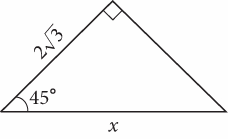p>In the figure above, what is the value of x?

• A.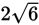• B. 6
• C.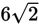• D. 8

Getting to the Answer: The measure of the third angle is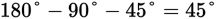, so the triangle is a 45-45-90 triangle. The side lengths of a 45-45-90 triangle are always in the ratio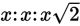, so the hypotenuse (which happens to be x in this question) is the length of a leg, multiplied by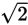. This means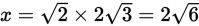, which is (A).